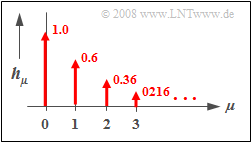# Digital Filters

## General block diagram

Each signal  $x(t)$  can be represented on a computer only by the sequence  $〈x_ν〉$  of its samples,  where  $x_ν$  stands for  $x(ν · T_{\rm A})$.

• To capture the influence of a linear filter with frequency response  $H(f)$  on the discrete-time signal  $〈x_ν〉$,  it makes sense to also describe the filter in discrete time.
• On the right you can see the corresponding block diagram.

Thus,  for the samples of the output signal applies:

$$y_\nu = \sum\limits_{\mu = 0}^M {a_\mu } \cdot x_{\nu - \mu } + \sum\limits_{\mu = 1}^M {b_\mu } \cdot y_{\nu - \mu } .$$

The applet  "Digital Filters" illustrates the subject matter of this chapter.
The following should be noted here:

• The first sum describes the dependence of the current output  $y_ν$  on the current input  $x_ν$  and on the  $M$  previous input values  $x_{ν–1}$, ... , $x_{ν–M}.$
• The second sum characterizes the influence of  $y_ν$  by the previous values  $y_{ν–1}$, ... , $y_{ν–M}$  at the filter output.  Thus,  it indicates the recursive part of the filter.
• The integer parameter  $M$  is called the »order«  of the digital filter.

## Non-recursive filter

$\text{Definition:}$  If all feedback coefficients are  $b_{\mu} = 0$, we speak of a  »non-recursive filter«.  Otherwise,  the filter is  "recursive".

Such a  $M$–th order non-recursive filter has the following properties:

• The output value  $y_ν$  depends only on the current and the  $M$  previous input values:
$$y_\nu = \sum\limits_{\mu = 0}^M {a_\mu \cdot x_{\mu - \nu } } .$$
• The filter impulse response is obtained from this with  $x(t) = δ(t)$:
$$h(t) = \sum\limits_{\mu = 0}^M {a_\mu \cdot \delta ( {t - \mu \cdot T_{\rm A} } )} .$$
• The corresponding input signal in discrete-time notation is:   $x_ν ≡0$  except for  $x_0 =1$.
• By applying the shifting theorem,  it follows for the filter frequency response:
$$H(f) = \sum\limits_{\mu = 0}^M {a_\mu \cdot {\rm{e}}^{ - {\rm{j}}\hspace{0.05cm} \cdot \hspace{0.05cm}2{\rm{\pi }}\hspace{0.05cm} \cdot \hspace{0.05cm}f \hspace{0.05cm} \cdot \hspace{0.05cm} \mu \hspace{0.05cm} \cdot \hspace{0.05cm} T_{\rm A} } } .$$

$\text{Example 1:}$  A two-way channel, where

• the signal arrives on the main path unattenuated with respect to the input signal,  but delayed by  $2\ \rm µ s$,  and
• is followed at a distance of  $4\ \rm µ s$  – i.e. absolutely at time  $t = 6\ \rm µ s$  – by an echo with half amplitude,

can be simulated by a non-recursive filter according to the above diagram, where the following parameter values are to be set:

$$M = 3,\quad T_{\rm A} = 2\;{\rm{µ s} },\quad a_{\rm 0} = 0,\quad a_{\rm 1} = 1, \quad a_{\rm 2} = 0, \quad a_{\rm 3} = 0.5.$$

## Recursive filter

$\text{Definition:}$  If all forward coefficients are  $a_\nu \equiv 0$  with the exception of  $a_0$,   then a  »(purely) recursive filter«  is present.

In the following,  we restrict ourselves to the special case  $M = 1$  (block diagram corresponding to the figure).  This filter has the following properties:

• The output value  $y_ν$  depends (indirectly) on an infinite number of input values:
$$y_\nu = \sum\limits_{\mu = 0}^\infty {a_0 \cdot {b_1} ^\mu \cdot x_{\nu - \mu } .}$$
• This is shown by the following calculation:
$$y_\nu = a_0 \cdot x_\nu + b_1 \cdot y_{\nu - 1} = a_0 \cdot x_\nu + a_0 \cdot b_1 \cdot x_{\nu - 1} + {b_1} ^2 \cdot y_{\nu - 2}.$$

$\text{Definition:}$

• The  »discrete-time impulse response«  $〈\hspace{0.05cm}h_\mu\hspace{0.05cm}〉$  is by definition the output sequence when a single  »one«  is present at the input at  $t =0$.
• For a recursive filter,  the discrete-time impulse response extends to infinity already with  $M = 1$:
$$h(t)= \sum\limits_{\mu = 0}^\infty {a_0 \cdot {b_1} ^\mu \cdot \delta ( {t - \mu \cdot T_{\rm A} } )}\hspace{0.3cm} \Rightarrow \hspace{0.3cm}〈\hspace{0.05cm}h_\mu\hspace{0.05cm}〉= 〈\hspace{0.05cm}a_0, \ a_0\cdot {b_1}, \ a_0\cdot {b_1}^2, \ a_0\cdot {b_1}^3, \ \text{...} \hspace{0.05cm}〉.$$

Further, it should be noted:

• For stability reasons,   $b_1 < 1$  must hold.
• If  $b_1 = 1$,  the impulse response  $h(t)$  would extend to infinity and if  $b_1 > 1$,    $h(t)$  would even resonate to infinity.
• In such a first-order recursive filter,  each individual Dirac delta line is smaller than the previous Dirac delta line by exactly the factor  $b_1$:
$$h_{\mu} = h(\mu \cdot T_{\rm A}) = {b_1} \cdot h_{\mu -1}.$$Discrete-time impulse response
of a recursive digital filter

$\text{Example 2:}$  The diagram on the right shows the discrete-time impulse response  $〈\hspace{0.05cm}h_\mu\hspace{0.05cm}〉$  of a first-order recursive filter with the parameters  $a_0 = 1$  and  $b_1 = 0.6$.

• The progression is exponentially decreasing and extends to infinity–in–time.
• The ratio of the weights of two successive Dirac delta lines is  $b_1 = 0.6$  in each case.